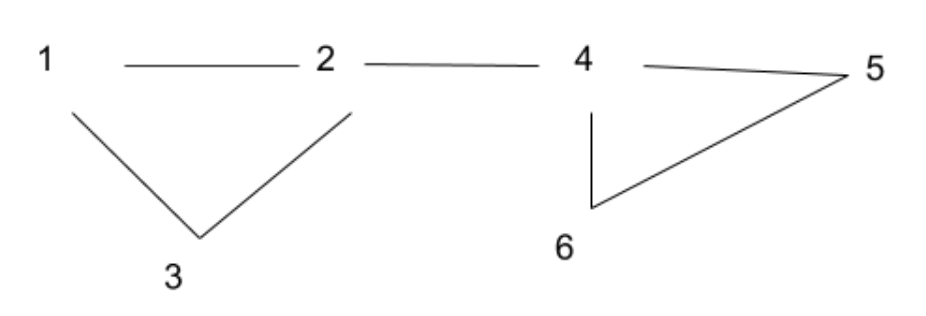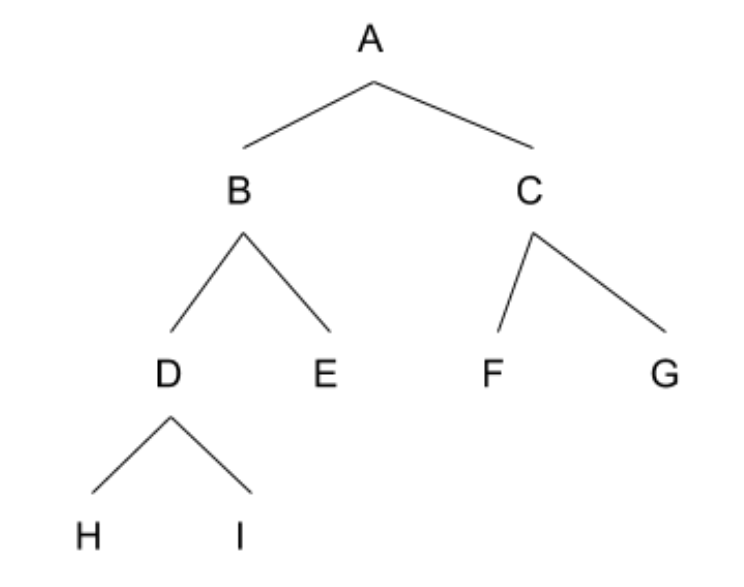# Nutanix Interview Experience 2018

Round 1: Coding Round [Hackerrank] – 60 Mins – 2 Ques

Q1: Given a set of mutual friends in a class, can you divide the class in two groups such that:
For all students in a group, each student is a friend of every other student?

Note: Friendship is not transitive, i.e. if A and B are friends, and B and C are friends, it does not imply that A and C are friends.

A group having a single student is a valid group in itself.

Input:
First line of input will contain t: number of test cases

First Line of each test case will contain two inputs N, M.
N nodes and M relations.

Next M lines contains two nodes A, B.
A B means A and B are friends.

Output: YES if you can, NO if no such division possible.

Example:
1
6 7
1 2
2 3
3 1
2 4
4 5
5 6
6 4
Output of this: TRUE1-2-3 is fully connected subgraph.
4-5-6 is fully connected subgraph.

Q2: Given a carpet of size a*b [length*breadth] and a box of size c*d, one has to fit the carpet in box in minimum number of moves. A move is to fold the carpet in half, either by length or breadth.

One can even turn the carpet by 90 degrees any number of times, won’t be counted as a move.

Example:
Box = 6 * 10
Carpet = 8 * 12

Output: No of moves = 1

Fold the carpet by breadth, 12/2
so now carpet is 6*8 and can fit fine.

Approach:
Try thinking of comparing smaller numbers and larger numbers. and just dividing the bigger number by 2, and rotating as many times needed.

Results: The cutoff of this round was passing at least 7/8 test cases of Q2. No one was able to pass all the test cases of Q1.

5 of us proceeded to the next round.

Round 2: Debugging Round [Pen and Paper] – 50 Mins

A C Code of Infix to Postfix Conversion was provided, 90-100 lines of code.

Basic layout of code:

 `struct` `stack_node { ` `    ``// using linked-list struct for stack nodes ` `    ``// this one seemed fine, no bugs ` `    ``// needed to be taken care of ` ` `  `    ``push() ``// insert at the end approach used here ` `        ``// changed the whole function to use ` `        ``// insert at the beginning approach ` `        ``// even malloc wasn’t used for new nodes ` ` `  `        ``pop() ``// deletion from the end ` `        ``// used deletion from front ` `        ``// free wasn’t used in deletion ` ` `  `        ``front() ``// used to get value of top of node ` `        ``// no issues here ` ` `  `        ``bool` `precedence() ``// had some issues in parameter ` `    ``// checks, swapped the parameters ` `}; ` `main() ` `{ ` ` `  `    ``struct` `node* head; ``// isn’t null initialised ` `    ``// make sure not to use nullptr, as it’s C ` ` `  `    ``int` `i = 0, j = 0; ` `    ``for` `(i = 0; i < ``strlen``(infixStr); i++) { ` `        ``if` `(infixStr[i] >= ‘A’ && infixStr <= ‘Z’) ` `            ``postfix[j] = infixStr[i]; ``// j++ needed here ` ` `  `        ``do` `{ ` `            ``char` `stackTop = front(head); ` `            ``// no check was done for head == null, ` `            ``// either here or in front () ` ` `  `            ``pop(head); ` `            ``// didn’t needed pop at the very first ` `            ``// peek of stack top ` ` `  `            ``if` `(precedence(infixStr[i], stackTop)) { ` `                ``push(infixStr[i], head); ` `                ``break``; ` `            ``} ` `            ``else` `{ ` `                ``postfix[j] = infixStr[i]; ` ` `  `                ``// many things need to be corrected here ` `                ``// pop ( ) needs to be called here ` `                ``// the postfix j++ bug again ` `                ``// postfix [j++] = stackTop, rather than infixStr ` `            ``} ` `        ``} ``while` `(1); ` ` `  `        ``printf``(“% s”, postFix); ``// missed adding a ‘\0’ terminator here ` `    ``} ` `} ` `// This is just a gist of the actual code `

Results:

3 out of 5 proceeded to the next round.

Round 3: Interview Round [Face to Face] – 45 Mins
Candidate-1:
Started with Resume.
Discussions on previous job [ had a 1 year experience before M.Tech ].
To-and-Fro on what challenges faced as per multiple processors or multi-threads.

Explained a bug where malloc initiated system lock and some other signal tried to acquire the lock, a race condition. And the methodology of how to find the source of the problem and asynchronous tasks at the core of it.

The interviewer seemed to be happy with it.

Only one question was to be discussed in this round.

Given a binary tree and a number X, find:
all the nodes at a distance X from root
all the nodes at a distance X from leaf nodes

Example:
Graph:
1
1 1
1 1 1 1
1 1 0 0 0 0 0 0
and X = 2.

Pictorially the graph looked like:Output:

Nodes at Distance 2 from Root: 4 [D E F G]
Nodes at Distance 2 from Leaf: 2 [ A and B]

Follow-Up:

Part A of the Question was simple.

For Part B:
Spent time explaining the different approaches to the solution (starting with Exponential, then O(n^2)).
20-25 mins had been gone till this point.

Then tried defining structures for creating a graph and using properties of BFS [adjacency list structures].
The interviewer stopped me there only and suggested thinking of a different solution, where we don’t even want to create a tree.

Since we got the input as a complete binary tree [ as 0’s and 1’s ]
So formulated a formula for finding nodes.
Formulated a simple solution from it
( if a leaf is at input[i][j] position, then it’s resulting nodes would be at
input [i] [j / (2^X)].

Now here comes the tricky part:
Interviewer asked me if the resulting solution already contains node “A”, then devise a technique so that we don’t see A again and again.

This was sort of a small but an impactful performance improvement.
After a few minutes of discussion, gave an approach where we need not look
input [i] [ j – 2^K] nodes, as they’d give same answer (defined a structure of storing leafs in right to left, and bottom to up manner, keeping count of 0’s in each row).

The interviewer asked me to code it up, but I didn’t got much time to do it (maybe he was using a stopwatch or something). Wrote partial code and time’s up.

RESULT: SELECTED

Candidate-2:

Started with a brief introduction about myself.
The same problem (binary tree) was given. I was supposed to write full code including taking input. But I asked if pseudo code would do and he agreed. I did a simple BFS for part (a) and DFS for part (b), an O(n) solution, and he was happy with it. Asked me to optimise once I was done with the code.
Discussed a few other questions on my resume later, but seems it was only because I finished early with the code. Asked about my learning expectations at Nutanix, and about problems I faced while doing one of the projects I had mentioned in my resume.

RESULT: SELECTED

Candidate-3:

Same problem was also given to me. I was asked not to write pseudo code and was asked to write the code in python. I was able to solve the first question and wrote the code. I also solved the second problem but struggled with writing code. After around 45-50 min the interviewer stopped me.
Later I was asked couple of questions about my resume and previous internship(2-3 min)

RESULT: NOT SELECTED

Write your Interview Experience or mail it to contribute@geeksforgeeks.orgMy Personal Notes arrow_drop_upCheck out this Author's contributed articles.

If you like GeeksforGeeks and would like to contribute, you can also write an article using contribute.geeksforgeeks.org or mail your article to contribute@geeksforgeeks.org. See your article appearing on the GeeksforGeeks main page and help other Geeks.

Please Improve this article if you find anything incorrect by clicking on the "Improve Article" button below.

Article Tags :
Practice Tags :

3

Please write to us at contribute@geeksforgeeks.org to report any issue with the above content.